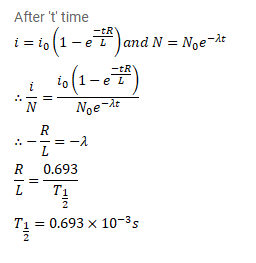# Radioactive isotopes are produced in a nuclear physics experiment at a constant rate

Question:

Radioactive isotopes are produced in a nuclear physics experiment at a constant rate $\mathrm{dN} / \mathrm{dt}=\mathrm{R}$. An inductor of inductance $100 \mathrm{mH}$, a resistance $100 \Omega$ and a battery are connected to form a series circuit. The circuit is switched on at instant the production of radioactive isotope starts. It is found that $\mathrm{i} / \mathrm{N}$ remains constant in time where $i$ is the current in the circuit at time $t$ and $N$ is the number of active nuclei at time t. Find the half-life of the isotopes.

Solution: